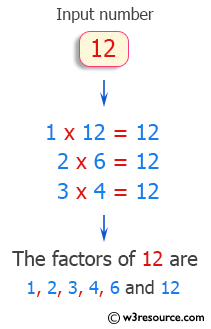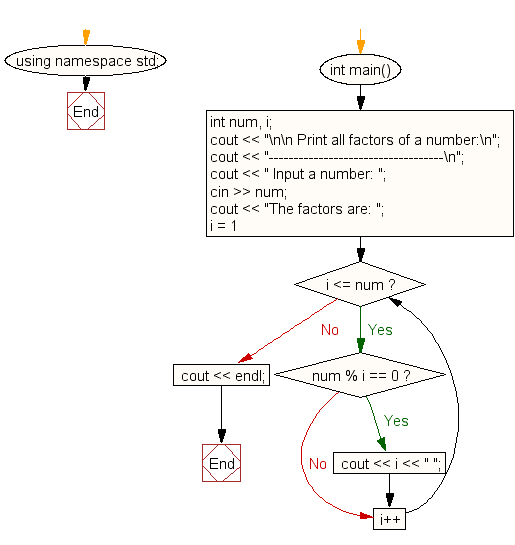﻿ C++ Exercises: Print all factors of the number - w3resource

# C++ Exercises: Print all factors of the number

## C++ For Loop: Exercise-63 with Solution

Write a program in C++ to enter any number and print all factors of the number.

Pictorial Presentation:Sample Solution:-

C++ Code :

``````#include <iostream>
using namespace std;
int main()
{
int num, i;
cout << "\n\n Print all factors of a number:\n";
cout << "-----------------------------------\n";
cout << " Input a number: ";
cin >> num;
cout << "The factors are: ";
for (i = 1; i <= num; i++)
{
if (num % i == 0)
{
cout << i << " ";
}
}
cout << endl;
}
``````

Sample Output:

``` Print all factors of a number:
-----------------------------------
Input a number: 63
The factors are: 1 3 7 9 21 63
```

Flowchart:C++ Code Editor: